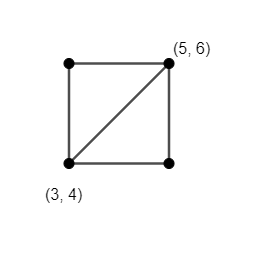Courses
Courses for Kids
Free study material
Free LIVE classes
MoreLIVE
Join Vedantu’s FREE Mastercalss

# Find the area of the square whose one pair of opposite vertices are (3,4) and (5,6).Verified
359.7k+ views
Hint: Calculate the length of diagonal of the square using distance formula between two points. Use the formula for calculating the area of square when the length of diagonal is given, which is $\dfrac{{{a}^{2}}}{2}$ where ‘a’ is the length of the diagonal. You can also calculate the length of the side of the square when a diagonal is given and then calculate the area of the square.

We have the coordinates (3,4) and (5,6) of two opposite vertices of the square. We have to find the area of the given square. To find the area, we will firstly calculate the length of the diagonal of the square using distance formula between two points.We know that distance between two points of the form $\left( {{x}_{1}},{{y}_{1}} \right)$ and $\left( {{x}_{2}},{{y}_{2}} \right)$ is $\sqrt{{{\left( {{x}_{1}}-{{x}_{2}} \right)}^{2}}+{{\left( {{y}_{1}}-{{y}_{2}} \right)}^{2}}}$.
Substituting ${{x}_{1}}=3,{{y}_{1}}=4,{{x}_{2}}=5,{{y}_{2}}=6$ in the above formula, the distance between the points (3,4) and (5,6) is $\sqrt{{{\left( 3-5 \right)}^{2}}+{{\left( 4-6 \right)}^{2}}}=\sqrt{{{2}^{2}}+{{2}^{2}}}=\sqrt{4+4}=\sqrt{8}=2\sqrt{2}$ units.
We will now evaluate the area of the square using the length of the diagonal of the square. We can also calculate the area of the square by finding the length of the edge of the square.
We know that the area of a square when the length of the edge is ‘a’ is ${{a}^{2}}$. So, the length of the diagonal is $\sqrt{2}a$.
If we substitute $x=\sqrt{2}a$, the area of the square will be $\dfrac{{{x}^{2}}}{2}$.
Substituting $x=2\sqrt{2}$, the area of square $=\dfrac{{{a}^{2}}}{2}=\dfrac{{{\left( 2\sqrt{2} \right)}^{2}}}{2}=\dfrac{8}{2}=4$ sq. units.
Hence, the area of the square whose two opposite vertices are (3,4) and (5,6) is 4 sq. units.

Note: We can also solve this question by finding the length of the edge of the square using the length of diagonal of the square and then using it to calculate the area of the square. We can also solve this question by finding other two coordinates of the square and then finding the area of the square using the formula for calculating the area of a polygon when vertices are given.

Last updated date: 23rd Sep 2023
Total views: 359.7k
Views today: 7.59k